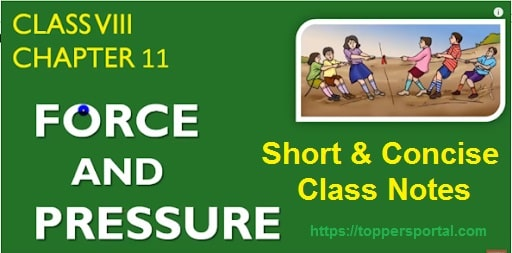## Force and Pressure Class 8 Notes Pdf DownloadFirstly, let us see what we are going to study in this chapter. The overview of the chapter is very necessary for every students. This gives you the idea about the content we are going to study and can relate with our previous syllabus or chapters.

Contents

Overview: Force and Pressure

Let us firstly see what we are going to study-

• What is Force
• Effects of Forces
• Types of Forces: Contact Forces and Non-Contact Forces
• Muscular Force
• Gravitational Force
• Electrostatic Force
• Magnetic Force
• Frictional Force
• Types of Frictional Force
• Air Resistance
• What is Pressure
• Mathematical Formula of Pressure
• Pressure exerted by Liquids and Gases
• Atmospheric Pressure

Force and Pressure Class 8 Notes [Short and Concise]

### What is Force?

In simple words, Force is a push or pull.

• Mathematically, Force = Mass × Acceleration
• The SI unit of Force is Newton. It is denoted by the symbol N (Capital ‘N’) after the name of Issac Newton.

### Effects of Forces

• Force can move an object or bring an object in the state of motion from the rest position.
• Force can stop an object or bring an object at rest from the state of motion
• Force can change the direction of an object
• Force can accelerate (speed up) or de-accelerate (slow down) an object
• Force can change the shape of an object
• Force can change the size of an object

### Types of Forces

There are two types of forces-

1. Contact Force
2. Non-Contact Force

#### Contact Forces

The type of forces experienced by bodies when they are in direct contact with each other.

Examples:

#### Non-Contact Forces

The type of forces which are able to push or pull even when the objects/bodies are not in physical or direct contact with each other.

Examples: The Forces that come under this category are-

### Electrostatic Force

The force applied by a charged body on another charged or uncharged body is called electrostatic force.

Like Charges repel each other and Unlike charges attract each other.

Like Charges: +ve and +ve or -ve and -ve

Unlike Charges: +ve and -ve

### Gravitational Force

It is the force of attraction that is acting between the objects in the universe.

Note: It is an attractive force

What is Gravity?

It is the gravitational pull of earth on the objects lying on or near the earth’s surface.

### Magnetic Force

The force attraction or repulsion produced by magnets. When magnetic materials are brought in near a magnet it attracts the objects.

Like Poles repel each other and Unlike Poles attract each other.

### Muscular Force

The forces due to the action of our muscles is called Muscular force.

### Frictional Force

#### What is Force of Friction (Frictional Force)?

It is the force that comes into action when the surfaces of two bodies come in contact with each other and it opposes the motion of the objects. It is not a fundamental force.

#### Types of Frictional Force

There are mainly two types of Frictional Forces-

1. Static Friction
2. Kinetic Friction (Sliding Friction & Rolling Friction)

## Pressure

### What is Pressure?

It is the force acting on the unit surface of an area.

Mathematically, Pressure = Force/Area

The SI Unit of pressure is N/m².

Question: What is the SI Unit of pressure?

(A) N/m

(B) pascal

(C) both A and B

(D) None of these

The correct answer is option (C) i.e., Both (A) and (B)

### Pressure Exerted by Liquids

Important Points:

• pressure exerted by liquid increases with depth
• pressure exerted by liquid is same in all directions at a given depth.

Manometer: It is an instrument used to measure the pressure difference

### Atmospheric Pressure

The pressure exerted by the weight of the atmosphere on the earth’s surface.

• 1 atm = 790 mmHg at sea level
• 1 atm = 101325 Pa.
• Atmospheric Pressure can be measured by barometer